Olympiad Test : Algebra - 2

# Olympiad Test : Algebra - 2

Test Description

## 20 Questions MCQ Test Maths Olympiad Class 6 | Olympiad Test : Algebra - 2

Olympiad Test : Algebra - 2 for Class 6 2022 is part of Maths Olympiad Class 6 preparation. The Olympiad Test : Algebra - 2 questions and answers have been prepared according to the Class 6 exam syllabus.The Olympiad Test : Algebra - 2 MCQs are made for Class 6 2022 Exam. Find important definitions, questions, notes, meanings, examples, exercises, MCQs and online tests for Olympiad Test : Algebra - 2 below.
 1 Crore+ students have signed up on EduRev. Have you?
Olympiad Test : Algebra - 2 - Question 1

### What is the value of x? 3(x + 6) + 2 (x + 3) = 64

Detailed Solution for Olympiad Test : Algebra - 2 - Question 1

3(x + 6) + 2(x + ) = 64
⇒ 3x + 18 + 2x + 6 =64
⇒ 5x + 24 = 64
⇒ 5x = 64 - 24
⇒ 5x = 40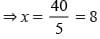Olympiad Test : Algebra - 2 - Question 2

### What is the value of P if 3(2 – 5P) – 2 (1 – 6P) = 1?

Detailed Solution for Olympiad Test : Algebra - 2 - Question 2

Here, 3(2 – 5p) –2 (1 – 6p) = 1
⇒ 6 - 15p - 2 + 12p =1
⇒ 4 - 3p = 1 ⇒ 3p = 4 -1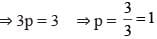Olympiad Test : Algebra - 2 - Question 3

### If 8 is subtracted from three times a number the result is 13. What is number?

Detailed Solution for Olympiad Test : Algebra - 2 - Question 3

Let the number be x.
∴ 3x - 8 = 13 ⇒ 3x = 13 + 8
⇒ 3x = 21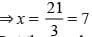Olympiad Test : Algebra - 2 - Question 4

The sum of three consecutive natural numbers is 114. Which is the greatest number?

Detailed Solution for Olympiad Test : Algebra - 2 - Question 4

Let the number are x, x + 1, x+ 2.
∴ x + x 1 + x + 2 = 114
⇒ 3x +3 = 114 ⇒ 3x = 114 - 3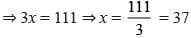Greatest number = 37 + 2 = 39

Olympiad Test : Algebra - 2 - Question 5

The length of a rectangular field is 5m more than its breadth. If the perimeter of the field is 74 m what is its length?

Detailed Solution for Olympiad Test : Algebra - 2 - Question 5

Let the breadth be x m.
Length = (x + 5)
Perimeter of rectangle = 74
⇒ 2(x + 5 + x) = 74
⇒ 2x + 5 = 37 ⇒ 2x = 37 - 5
⇒ 2x = 32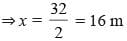Length = 16 + 5 = 21m

Olympiad Test : Algebra - 2 - Question 6

If 9 is added to twice of a certain number, the result is 57, what is that number?

Detailed Solution for Olympiad Test : Algebra - 2 - Question 6

Let the number be x.
2x + 9 = 57 ⇒ 2x = 57– 9 = 48
x = 24

Olympiad Test : Algebra - 2 - Question 7

If 7 is subtracted from a number, we get 37, then what is that number?

Detailed Solution for Olympiad Test : Algebra - 2 - Question 7

Let the number be x.
∴ x - 7 = 37 ⇒ x = 37 + 7 = 44

Olympiad Test : Algebra - 2 - Question 8

A man is thrice as old as his daughter. 5 years ago the man was four times as old as his  daughter, what is the age of the daughter?

Detailed Solution for Olympiad Test : Algebra - 2 - Question 8

Let the age of daughter be x years.
∴ Man's age = 3x.
and 5 years ago, daughter's age = x-5
∴ Man's age = 3x —5
⇒ 3x —5= 4x —20 = 4x —3x = — 5 + 20
⇒ x =15 years

Olympiad Test : Algebra - 2 - Question 9

Karan’s father is thrice as old as Karan. After 14 years his age will be twice that of his son. What is the age of Karan?

Detailed Solution for Olympiad Test : Algebra - 2 - Question 9

Let the age of karan be x years.
Father’s age = 3x years
After 14 years,
3x +14 = 2(x –14)
⇒ 3x + 14 = 2x + 28
⇒ x = 28 - 14
⇒ x = 14 years

Olympiad Test : Algebra - 2 - Question 10

The sum of three consecutive even numbers is 78. Which number is smallest among them?

Detailed Solution for Olympiad Test : Algebra - 2 - Question 10

Let the three consecutive even numbers are
x, x + 2, x + 4.
∴ x + x + 2 + x + 4 =78
⇒ 3x = 78 - 6
⇒ 3x = 72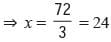Olympiad Test : Algebra - 2 - Question 11

Five times the price of a radio is Rs 170 more than three times its price. What is the price of the radio?

Detailed Solution for Olympiad Test : Algebra - 2 - Question 11

Let the price of the ratio be Rs x.
5x =170 + 3x
⇒ 2x =170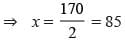Olympiad Test : Algebra - 2 - Question 12

The length of a rectangular park is thrice its breadth. If the perimeter of the park is 168m, what is its breadth?

Detailed Solution for Olympiad Test : Algebra - 2 - Question 12

Let the breadth be x m
Length = 3xm
Perimeter of rectangular park = 168Olympiad Test : Algebra - 2 - Question 13

Kiran multiplies a certain number by 17 and adds 4 to the product, she gets 225. What is that number?

Detailed Solution for Olympiad Test : Algebra - 2 - Question 13

Let the number be x.
∴ x × 17 + 4 = 225
⇒ 17x = 225 - 4
⇒ 17x = 221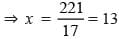Olympiad Test : Algebra - 2 - Question 14

What is the sum of the expressions 3a – 2b + 5c, 2a + 5b – 7c and –a – b + c?

Detailed Solution for Olympiad Test : Algebra - 2 - Question 14

Required result
= 3a –2b + 5c + 2a +5b –7c – a – b + c
= 4a + 2b – c

Olympiad Test : Algebra - 2 - Question 15

What is the co-efficient of x3 in 4y2zx3?

Olympiad Test : Algebra - 2 - Question 16

What is the value of 2a – [3b –{a – (2c – 3b) + 4c – 3 (a – b – 2c)}]

Detailed Solution for Olympiad Test : Algebra - 2 - Question 16

Required result
= 2a–[3b–{a – (2c + 3b) + 4c –3a + 3b + 6c}]
= 2a –[3b–{–2a +6b +8c}]
= 2a –[3b +2a – 6b – 8c]
= 2a –[2a–3b–8c] = 2a –2a + 3b +8c
= 3b + 8c

Olympiad Test : Algebra - 2 - Question 17

What is the value of xy – [yz – zx –{yx – (3y – xz) – (xy – zy)}]?

Detailed Solution for Olympiad Test : Algebra - 2 - Question 17

xy – [ yz – zx – {yx – (3y – xz) – (xy – zy)}]
=  xy – [ yz – zx – {yx – 3y + xz– xy + zy}]
=  xy – [ yz – zx – yx + 3y – xz + xy – zy]
=  xy + 2xz –3y

Olympiad Test : Algebra - 2 - Question 18

What is the value of x?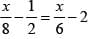Detailed Solution for Olympiad Test : Algebra - 2 - Question 18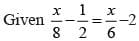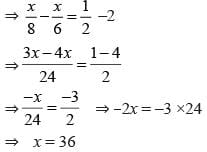Olympiad Test : Algebra - 2 - Question 19

If a number is tripled and the result is increased by 7, we get 70, then what is the number?

Detailed Solution for Olympiad Test : Algebra - 2 - Question 19

Let the number be x.
∴ 3 × x + 7 = 70
⇒ 3x = 70 - 7
⇒ 3x = 63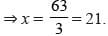Olympiad Test : Algebra - 2 - Question 20

A wire of length 86cm is bent in the form of a rectangle such that its length is 7 cm more than its breadth. What is its length ?

Detailed Solution for Olympiad Test : Algebra - 2 - Question 20

∴ Length = x + 7
Perimeter of wire = 86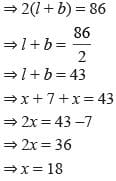∴ Length = x + 7 = 18 + 7 = 25 cm